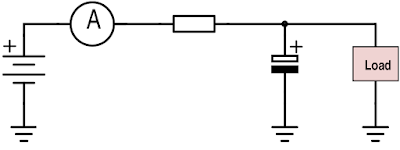## Wednesday, August 26, 2015

### Measuring average current of low power devices

Low power devices often spend most of their time in some sort of sleep mode, in which the power supply current is very low (some hundreds of nanoamperes typical). However, at times they wake up and can draw very large currents for a short period of time. The average current of such devices is often of interest, for example to estimate battery life.

Measuring the current directly is infeasible, due to the very large current range as well as the short duration of the high current pulses. A simple solution however is to measure the current through a RC filter circuit as shown in figure 1.Figure 1. Measurement setup.

There are a couple of things to consider though when doing this.
• The time constant of the RC filter needs to be much longer than the measurement period of the multimeter used
• Electrolytic capacitors initially exhibit large non-constant leakage currents
• Resistive voltage drop
A nice trick is to use the measurement resistor of the multimeter as the R of the circuit and to choose the capacitance then accordingly. My UT61E updates twice per second and has a measurement resistance of 1 kilo-ohm in the microampere range. I've used a capacitance of around 15000 uF, which gives a time constant of 15 seconds. The time constant shouldn't be too long either, as it makes the required measurement time longer.

The leakage current of electrolytic capacitors may require a long time to stabilize. After 24 hours of operation my bank of 15000 uF still had 500 nA of leakage, but decreased to around 40 nA after 48 hours. Constant leakage currents due to the measurement setup are simply subtracted from the measured average current.

I've never had a problem with any significant voltage drops with this setup. However, the voltage drops can be made smaller by increasing the capacitance. Notice that the average voltage seen by the load is still dependent on the average current and the resistance.